# Experimental design¶

Data

• Allows us to answer questions

• Need rigorous methods

Approach

• Build hypotheses with exploratory data analysis
• Test hypotheses with statistical tests
In :
import pandas as pd
import numpy as np
import matplotlib.pyplot as plt
import seaborn as sns
sns.set(style="darkgrid", color_codes=True)

In :
People = pd.read_csv('weight-height.csv')
People.info()

<class 'pandas.core.frame.DataFrame'>
RangeIndex: 10000 entries, 0 to 9999
Data columns (total 3 columns):
Gender    10000 non-null object
Height    10000 non-null float64
Weight    10000 non-null float64
dtypes: float64(2), object(1)
memory usage: 234.5+ KB

In :
People.head()

Out:
Gender Height Weight
0 Male 73.847017 241.893563
1 Male 68.781904 162.310473
2 Male 74.110105 212.740856
3 Male 71.730978 220.042470
4 Male 69.881796 206.349801

# From observed pattern to reliable result¶

Data contains patterns

• Some expected
• Others surprising
• Random variation also

Dealing with this

• How do we go from observation to result?

## Two hypotheses¶

Null hypothesis (H0)

• A = B
• Observed patterns are the product of random chance

Alternative hypothesis (H1)

• A != B
• Difference between samples represents a real difference between the populations

### Some statistical terms¶

p-value

• The likelihood that the distribution of values would occur if the null hypothesis were correct. We cant be be 100% sure that are pattern couldn't have emerged due to random chance, but we can quantify the probability that random chance will produce a given pattern. The smaller the p-value the less likely it is that the null hypothesis can account for our observations.

alpha

• Crucial threshold of p-value
• Usually alpha < 0.05: reject null hypothesis

# Student's t-test¶

The t-test tells you how significant the differences between groups are; In other words it lets you know if those differences (measured in means or averages) could have happened by random chance.

Two basic types:

• One-sample: Mean of population different from a given value?
• Two-sample: Two population means equal?

## First t-test¶

We want to compare the mean heights in cm of the Sample_A group with a given value. We want to see whether the mean weight of the people in this sample is significantly different from the chosen cut-off point of 65 kg. We will use a one-sample t-test, which allows us to compare the mean of a sample with a chosen value. We will perform this test on the sample provided versus the crucial value of 65 kg, and test its significance by comparing the value of alpha to the p-value.

In :
# Get Sample
Sample_A = People.Height.values

plt.legend(('Height'))
plt.show()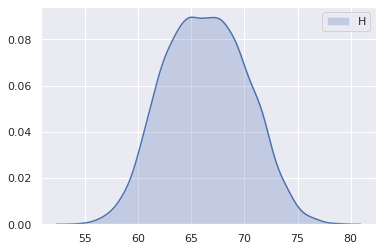In :
from scipy import stats

# Perform t-test and print result
t_result = stats.ttest_1samp(Sample_A, 65)

# Test significance
alpha = 0.05
if (t_result < alpha):
print(f"mean value of Sample A differs from given value \np-value: {t_result}")
else:
print(f"No significant difference found \np-value: {t_result}")

mean value of Sample A differs from given value
p-value: 1.089548024807223e-260


## Two-sample t-test¶

Now we'll compare two sets of samples. Again, we'll be looking at the weight (weight of males and females) but this time we're going to compare the weight of males with females. Does the mean weight differ between the two genders? A two-sample t-test can tell us whether the means of two samples differ significantly.

In :
# Get Sample
Sample_A = People[People['Gender']=='Male']['Weight']
Sample_B = People[People['Gender']=='Female']['Weight']

plt.legend(('Male Weight', 'Female Weight'))
plt.show()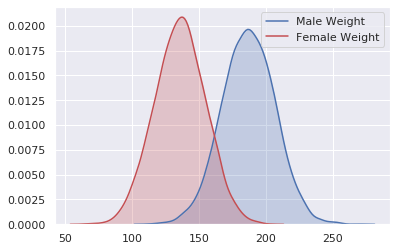In :
t_result = stats.ttest_ind(Sample_A, Sample_B)

alpha = 0.05
if (t_result < alpha):
print(f"mean value of Sample A differs from Sample_B \np-value: {t_result}")
else:
print(f"No significant difference found \np-value: {t_result}")

mean value of Sample A differs from Sample_B
p-value: 0.0


# Testing proportion¶

## Hypothesis tests¶

Chi-square:

• Examine proportions of discrete categories

Fisher exact test:

• Examine proportions of discrete categories

## Chi-Squared Test¶

• A chi-square goodness of fit test determines if a sample data matches a population.

• A chi-square test for independence compares two variables in a contingency table to see if they are related. In a more general sense, it tests to see whether distributions of categorical variables differ from each another.

• A small chi square test statistic means that your observed data fits your expected data (there is a relationship).
• A large chi square test statistic means that the data does not fit very well (there isn’t a relationship).
In :
# Categorical Dataset

Out:
age job marital education default balance housing loan contact day month duration campaign pdays previous poutcome y
0 58 management married tertiary no 2143 yes no unknown 5 may 261 1 -1 0 unknown no
1 44 technician single secondary no 29 yes no unknown 5 may 151 1 -1 0 unknown no
2 33 entrepreneur married secondary no 2 yes yes unknown 5 may 76 1 -1 0 unknown no
3 47 blue-collar married unknown no 1506 yes no unknown 5 may 92 1 -1 0 unknown no
4 33 unknown single unknown no 1 no no unknown 5 may 198 1 -1 0 unknown no

### Contingency Table¶

The table was called a contingency table, by Karl Pearson, because the intent is to help determine whether one variable is contingent upon or depends upon the other variable. For example, does an Education in primary, secondary, tertiary, or unknown depend on Job, or are they independent?

We can summarize the collected observations in a table with one variable corresponding to columns and another variable corresponding to rows. Each cell in the table corresponds to the count or frequency of observations that correspond to the row and column categories.

Historically, a table summarization of two categorical variables in this form is called a contingency table.

For example, the Education = rows and Job = columns table with contrived counts might look as follows:

In :
table = pd.crosstab(df.job, df.education)
table

Out:
education primary secondary tertiary unknown
job
blue-collar 3758 5371 149 454
entrepreneur 183 542 686 76
housemaid 627 395 173 45
management 294 1121 7801 242
retired 795 984 366 119
self-employed 130 577 833 39
services 345 3457 202 150
student 44 508 223 163
technician 158 5229 1968 242
unemployed 257 728 289 29
unknown 51 71 39 127

The Chi-Squared test is a statistical hypothesis test that assumes (the null hypothesis) that the observed frequencies for a categorical variable match the expected frequencies for the categorical variable. The test calculates a statistic that has a chi-squared distribution, named for the Greek capital letter Chi (X) pronounced ki as in kite.

Given the Job/Education example above, the number of observations for a category may or may not be the same. Nevertheless, we can calculate the expected frequency of observations in each Education group and see whether the partitioning of Education by Job results in similar or different frequencies.

The Chi-Squared test does this for a contingency table, first calculating the expected frequencies for the groups, then determining whether the division of the groups, called the observed frequencies, matches the expected frequencies.

The result of the test is a test statistic that has a chi-squared distribution and can be interpreted to reject or fail to reject the assumption or null hypothesis that the observed and expected frequencies are the same.

We can interpret the test statistic in the context of the chi-squared distribution with the requisite number of degress of freedom as follows:

• If Statistic >= Critical Value: significant result, reject null hypothesis (H0), dependent.
• If Statistic <= Critical Value: not significant result, fail to reject null hypothesis (H0), independent.

Degrees of Freedom:

• Degrees of Freedom (df) refers to the maximum number of logically independent values, which are values that have the freedom to vary, in the data sample or the number of independent pieces of information that went into calculating the estimate. It’s not quite the same as the number of items in the sample. In order to get the df for the estimate, you have to subtract 1 from the number of items. Let’s say you were finding the mean weight loss for a low-carb diet. You could use 4 people, giving 3 degrees of freedom (4 – 1 = 3), or you could use 100 people with df = 99.

The degrees of freedom for the chi-squared distribution is calculated based on the size of the contingency table as:

• degrees of freedom = (rows - 1) * (cols - 1)

In terms of a p-value and a chosen significance level (alpha), the test can be interpreted as follows:

• If p-value <= alpha: significant result, reject null hypothesis (H0), dependent.
• If p-value > alpha: not significant result, fail to reject null hypothesis (H0), independent.

For the test to be effective, at least five observations are required in each cell of the contingency table.

### Example Chi-Squared Test¶

The Pearson’s chi-squared test for independence can be calculated in Python using the chi2_contingency() SciPy function.

The function takes an array as input representing the contingency table for the categorical variables. It returns the calculated statistic and p-value for interpretation as well as the calculated degrees of freedom and table of expected frequencies.

In :
# chi-squared test with similar proportions
from scipy.stats import chi2_contingency
from scipy.stats import chi2

stat, p_val, dof, expected = chi2_contingency(table)
print(table)
print(f'\ndof = {dof}')

education      primary  secondary  tertiary  unknown
job
blue-collar       3758       5371       149      454
entrepreneur       183        542       686       76
housemaid          627        395       173       45
management         294       1121      7801      242
retired            795        984       366      119
self-employed      130        577       833       39
services           345       3457       202      150
student             44        508       223      163
technician         158       5229      1968      242
unemployed         257        728       289       29
unknown             51         71        39      127

dof = 33


We can interpret the statistic by retrieving the critical value from the chi-squared distribution for the probability and number of degrees of freedom.

For example, a probability of 95% can be used, suggesting that the finding of the test is quite likely given the assumption of the test that the variable is independent. If the statistic is less than or equal to the critical value, we can fail to reject this assumption (independent), otherwise it can be rejected (dependent).

In :
# interpret test-statistic
prob = 0.95

critical = chi2.ppf(prob, dof)
print(f'Probability = {prob}, Critical Value = {round(critical,3)}, Test Statistic = {round(stat,3)}')

if abs(stat) >= critical:
print('\nDependent (reject H0)')
else:
print('\nIndependent (fail to reject H0)')

Probability = 0.95, Critical Value = 47.4, Test Statistic = 28483.136

Dependent (reject H0)


We can also interpret the p-value by comparing it to a chosen significance level, which would be 5%, calculated by inverting the 95% probability used in the critical value interpretation.

In :
# interpret p-value
alpha = 1.0 - prob
print(f'Significance Level = {round(alpha,3)}, P-Value = {round(p_val,3)}')

if p_val <= alpha:
print('\nDependent (reject H0)')
else:
print('\nIndependent (fail to reject H0)')

Significance Level = 0.05, P-Value = 0.0

Dependent (reject H0)


## Fisher's exact test¶

Fisher's exact test is a statistical significance test used in the analysis of contingency tables. Although in practice it is employed when sample sizes are small, it is valid for all sample sizes. Use the Fisher's exact test of independence when you have two nominal (categorical) variables and want to find out if proportions for one nominal variable are different among values of the other nominal variable.

Test distinguishes between:

• Null hypothesis:
• Two samples have same distribution of outcomes
• Are these two discrete variables related?
• Alternative hypothesis:
• Two samples have different distribution of outcomes

Now, we will work with the Olympics dataset to look at the relative success of the American swimming and athletics teams. Whether each athlete received a medal is coded as True or False in the MedalTF column of athletes. Do a larger proportion of swimming or athletics participants come home with medals? A Fisher exact test is a useful way to compare proportions of samples falling into discrete categories. To test this, we will need to perform a Fisher exact test on MedalTF in relation to Sport.

In :
athletes = pd.read_csv('https://assets.datacamp.com/production/repositories/4371/datasets/8fd0a14bfbc5f13719d92334eaf77b23f2e914d6/olyathswim.csv')

Out:
Unnamed: 0 Name Sex Age Height Weight Team NOC Games Year City Sport Event Medal
0 27 Cornelia "Cor" Aalten (-Strannood) F 18.0 168.0 NaN Netherlands NED 1932 Summer 1932 Los Angeles Athletics Athletics Women's 100 metres NaN
1 28 Cornelia "Cor" Aalten (-Strannood) F 18.0 168.0 NaN Netherlands NED 1932 Summer 1932 Los Angeles Athletics Athletics Women's 4 x 100 metres Relay NaN
2 30 Einar Ferdinand "Einari" Aalto M 26.0 NaN NaN Finland FIN 1952 Summer 1952 Helsinki Swimming Swimming Men's 400 metres Freestyle NaN
3 36 Arvo Ossian Aaltonen M 22.0 NaN NaN Finland FIN 1912 Summer 1912 Stockholm Swimming Swimming Men's 200 metres Breaststroke NaN
4 37 Arvo Ossian Aaltonen M 22.0 NaN NaN Finland FIN 1912 Summer 1912 Stockholm Swimming Swimming Men's 400 metres Breaststroke NaN
In :
athletes['Medal'].unique()

Out:
array([nan, 'Bronze', 'Silver', 'Gold'], dtype=object)
In :
b = {'Bronze': True, 'Silver': True, 'Gold': True}
athletes['MedalTF'] = athletes['Medal'].map(b)
athletes['MedalTF'] = athletes['MedalTF'].fillna(False)
athletes['Medal'] = athletes['Medal'].fillna('None')
athletes = athletes.dropna()

In :
athletes['MedalTF'].unique()

Out:
array([False,  True])
In :
# Create a table of cross-tabulations
table = pd.crosstab(athletes.MedalTF, athletes.Sport)
print(table)

# Perform the Fisher exact test
fisher = stats.fisher_exact(table, alternative='two-sided')

# Is the result significant?
alpha = 0.05
if fisher <= alpha:
print(f"\nProportions of medal winners differ significantly \np-value: {fisher}")
else:
print(f"\nNo significant difference in proportions of medal winners found \np-value: {fisher}")

Sport    Athletics  Swimming
MedalTF
False        28726     16290
True          3648      2486

Proportions of medal winners differ significantly
p-value: 5.091646967990442e-11


According to these results, a higher proportion of swimmers come home with medals than athletics competitors.

# Random sampling¶

In this exercise, we're going to look at random sampling. For the purposes of this exercise, we are interested in differences between the body Weight of competitors in swimming and athletics. In order to test this, we will be using a two-sample t-test. However, we will be performing this test on a random sample of the data. By playing with the random subset chosen, we will see how randomness affects the results.

In :
# Define random seed
seed = 420

# Create two subsets, one for the athletics competitors and one for the swimmers
subsetathl = athletes[athletes.Sport == "Athletics"].dropna().sample(n=100, random_state = seed)
subsetswim = athletes[athletes.Sport == "Swimming"].dropna().sample(n=100, random_state = seed)

plt.legend(('Athletics Weight', 'Swimming Weight'))
plt.show()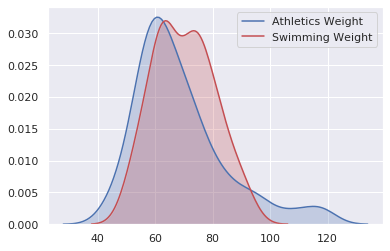In :
# Perform the two-sample t-test
t_result = stats.ttest_ind(subsetathl.Weight, subsetswim.Weight)
print(f'Test Statistic = {round(t_result,2)}, P-value = {round(t_result,2)}')

Test Statistic = -0.62, P-value = 0.54


Change the seed value to 1234 and re-run the code

In :
# Define random seed
seed = 1234

# Create two subsets, one for the athletics competitors and one for the swimmers
subsetathl = athletes[athletes.Sport == "Athletics"].dropna().sample(n=100, random_state = seed)
subsetswim = athletes[athletes.Sport == "Swimming"].dropna().sample(n=100, random_state = seed)

# Perform the two-sample t-test
t_result = stats.ttest_ind(subsetathl.Weight, subsetswim.Weight)
print(f'Test Statistic = {round(t_result,2)}, P-value = {round(t_result,2)}')

Test Statistic = 0.13, P-value = 0.9


Now we are familiar with randomizing samples and you can see how choosing different random samples can give you different results. Did you notice that our p-value changed when a different random sample was chosen? Improving our approach to create a balanced dataset would help avoid this problem.

### Blocking¶

Confounding variable

• Additional variable not accounted for in study design.
• Alters the independent and dependent variables.

Blocking = Solution to confounding

• Control for confounding by balancing with respect to other variable.

We're going to have another look at the same data but, this time, we'll use blocking to improve our approach. Like last time, we will be using a two-sample t-test on athlete Weight within the DataFrame, athletes. This time, however, we will control for Sex as a blocking factor, sampling equally from male and female participants. We will need to extract a random subset of athletes from both events to run our test.

In :
seed = 9000

# Create subset blocks
subsetathlm = athletes[(athletes.Sport == "Athletics") & (athletes.Sex == "M")].sample(n=100, random_state= seed)
subsetathlf = athletes[(athletes.Sport == "Athletics") & (athletes.Sex == "F")].sample(n=100, random_state= seed)
subsetswimm = athletes[(athletes.Sport == "Swimming") & (athletes.Sex == "M")].sample(n=100, random_state= seed)
subsetswimf = athletes[(athletes.Sport == "Swimming") & (athletes.Sex == "F")].sample(n=100, random_state= seed)

sns.boxplot(x="Sport", y="Weight", hue="Sex",
data=athletes);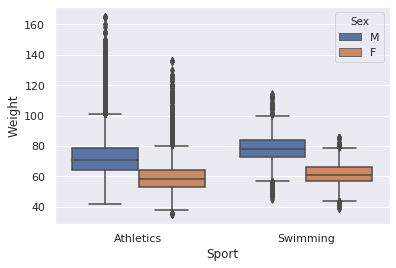In :
# Combine blocks
subsetathl = pd.concat([subsetathlm, subsetathlf])
subsetswim = pd.concat([subsetswimm, subsetswimf])

# Perform the two-sample t-test
t_result = stats.ttest_ind(subsetathl.Weight, subsetswim.Weight)

print(f'Test Statistic = {round(t_result,2)}, P-value = {round(t_result,2)}')

Test Statistic = -2.15, P-value = 0.03


Now our t-test is significant, with a p-value under 0.05. This blocked design has resolved the issue we had. You can see how this type of blocking approach can be a useful way to improve your experimental design when a confounding variable is present.

# Paired t-test¶

A paired t-test is used to determine whether the mean difference between two sets of observations is zero. In a paired sample t-test, each subject or entity is measured twice, resulting in pairs of observations.

• Controls for individual variation.
• Increases statistical power by reducing noise.

Here, we have a small DataFrame (podataframe) containing information on 10 Fields. We are interested in potato yield in tons/hectare. For each Field, we have a value for its Yield2018, before the application of a new fertilizer, and its Yield2019, after the application of the new fertilizer. We will perform two t-tests, a standard two-sample test, and a paired t-test. A paired t-test will control for the variation between fields.

In :
yields2018 = [60.2, 12, 13.8, 91.8, 50, 27, 71.1, 41, 31, 55.9]
yields2019 = [63.2, 15.6, 14.8, 96.7, 53, 29.9, 73, 44.4, 38, 57.3]

podataframe = pd.DataFrame({'Yield2018': yields2018,
'Yield2019' : yields2019})
print(podataframe)

   Yield2018  Yield2019
0       60.2       63.2
1       12.0       15.6
2       13.8       14.8
3       91.8       96.7
4       50.0       53.0
5       27.0       29.9
6       71.1       73.0
7       41.0       44.4
8       31.0       38.0
9       55.9       57.3

In :
sns.kdeplot(yields2018, shade=True, color="b")
plt.legend(('yields2018', 'yields2019'))
plt.show()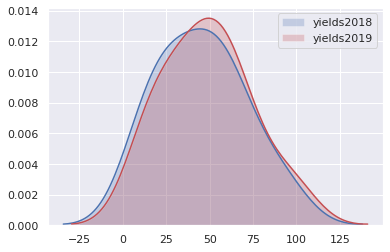In :
# Perform independent t-test
ttestind = stats.ttest_ind(podataframe.Yield2018, podataframe.Yield2019)

print(f'Test Statistic = {round(ttestind,2)}, P-value = {round(ttestind,2)}')

Test Statistic = -0.28, P-value = 0.78

In :
# Perform paired t-test
ttestpair = stats.ttest_rel(podataframe.Yield2018, podataframe.Yield2019)

print(f'Test Statistic = {round(ttestpair,2)}, P-value = {round(ttestpair,2)}')

Test Statistic = -5.81, P-value = 0.0


Recall, the paired test is more sensitive than the independent test and can pick up a difference that the independent test can't detect. This is because the difference within the samples each year (individual field effect) is quite large in comparison to the difference between the two years (effect of treatment). Paired tests are useful when a large variability exists.

# Analysis of variance ANOVA¶

Analysis of variance (ANOVA) is used to analyze the differences among group means in a sample. ANOVA provides a statistical test of whether two or more population means are equal, and therefore generalizes the t-test beyond two means.

ANOVA uses F-tests to statistically test the equality of means. F-statistics are based on the ratio of mean squares. It is simply an estimate of population variance that accounts for the degrees of freedom (DF) used to calculate that estimate.

Test types:

One Way ANOVA

• A one way ANOVA is used to compare two means from two independent (unrelated) groups using the F-distribution. The null hypothesis for the test is that the two means are equal. Therefore, a significant result means that the two means are unequal. A one way ANOVA will tell you that at least two groups were different from each other. But it won’t tell you which groups were different.

Two Way ANOVA

• The two-way ANOVA compares the mean differences between groups that have been split on two independent variables (called factors). The primary purpose of a two-way ANOVA is to understand if there is an interaction between the two independent variables on the dependent variable. For example, you could use a two-way ANOVA to understand whether there is an interaction between gender and educational level on test anxiety, where gender (males/females) and education level (undergraduate/postgraduate) are your independent variables, and test anxiety is your dependent variable.

## One-way ANOVA¶

Let's have another look at some data from our Olympic dataset. How does the Weight of athletes vary between teams from different countries? We going to use a one-way ANOVA to check for the presence of significant variation in Weight of Olympic athletes. A one-way ANOVA will allow us to see whether any differences between these groups of values are significant.

In :
sns.boxplot(x="Team", y="Weight",
data=athletes[athletes['Team'].isin(['United States','China', 'France'])]);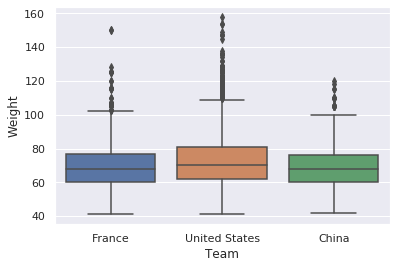In :
# Create arrays
France_athletes = athletes[athletes.Team == 'France'].Weight
US_athletes = athletes[athletes.Team == 'United States'].Weight
China_athletes = athletes[athletes.Team == 'China'].Weight

# Perform one-way ANOVA
anova = stats.f_oneway(France_athletes, US_athletes, China_athletes)
print(f'Test Statistic = {round(anova,2)}, P-value = {round(anova,3)}')

Test Statistic = 55.63, P-value = 0.0


According to this test, significant differences exist between the weights of athletes for each country.

## Two-way ANOVA¶

Let's have another look at some data from our Olympic dataset. How does the Weight of athletes vary between teams from different countries and of different sexes? We are going to use a two-way ANOVA to check for the presence of significant variation in the Weight of Olympic sprinters. A two-way ANOVA will allow you to see which of these two factors, Sex and Team, have a significant effect on Weight.

In :
sns.boxplot(x="Team", y="Weight", hue="Sex",
data=athletes[athletes['Team'].isin(['United States','China'])]);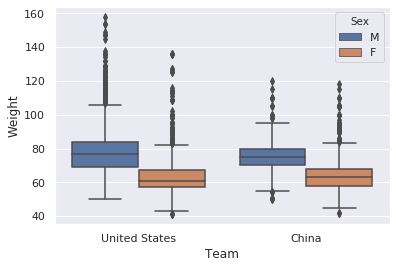In :
import statsmodels.api as sm

# Create model
formula = 'Weight ~ Sex + Team'
model = sm.formula.ols(formula, data=athletes).fit()

# Perform ANOVA and print table
aov_table = sm.stats.anova_lm(model, typ=2)
print(aov_table)

                sum_sq       df             F  PR(>F)
Sex       2.639780e+06      1.0  20144.562135     0.0
Team      9.344392e+05    228.0     31.275651     0.0
Residual  6.672648e+06  50920.0           NaN     NaN


According to the results of your ANOVA, Sex and Team has a significant effect.

# Interactive effects¶

Interaction effects represent the combined effects of factors on the dependent measure. When an interaction effect is present, the impact of one factor depends on the level of the other factor. Part of the power of ANOVA is the ability to estimate and test interaction effects. As Pedhazur and Schmelkin note, the idea that multiple effects should be studied in research rather than the isolated effects of single variables is one of the important contributions of Sir Ronald Fisher. When interaction effects are present, it means that interpretation of the main effects is incomplete or misleading. For example, the relationship between condiments and enjoyment probably depends on the type of food.

## Two-way ANOVA with interactive effects¶

Once again, we are going to look at our dataset of Olympic athletes. As in previous exercises, we will be looking at the variation in athlete Weight. We are going to look at athletes of either Sex competing in one of two Events: the 100 meter and 10,000 meter run. An ANOVA will allow us to work out which of these variables affect Weight and whether an interactive effect is present.

In :
df = athletes[athletes['Event'].isin(["Athletics Men's 10,000 metres",
"Athletics Men's 100 metres",
"Athletics Women's 10,000 metres",
"Athletics Women's 100 metres"])]

ax = sns.boxplot(data=df, x="Event", y="Weight", hue="Sex")
ax.set_xticklabels(['10,000m M','100m M','10,000m W','100m W']);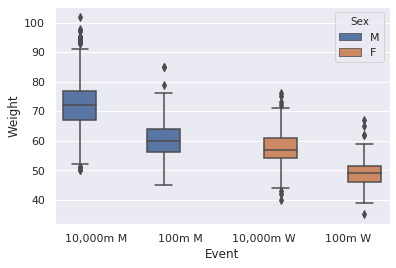In :
# Create model
formula = 'Weight ~ Sex + Event + Sex:Event'
model = sm.formula.ols(formula, data=athletes).fit()

# Perform ANOVA and print table
aov_table = sm.stats.anova_lm(model)
print(aov_table)

                df        sum_sq       mean_sq             F    PR(>F)
Sex            1.0  2.521601e+06  2.521601e+06  44267.620977  0.000000
Event        126.0  4.700899e+06  3.730872e+04    654.968041  0.000000
Sex:Event    126.0  9.832199e+03  7.803333e+01      1.369903  0.003758
Residual   51023.0  2.906406e+06  5.696266e+01           NaN       NaN


Look at the p-values for the effects of Sex, Event, and Sex:Event. Using a standard alpha of 0.05, Sex, Event, and Sex:Event have a significant effect on Weight. This means that both factors influence Weight, and the effect of one factor is dependent on the other.

## Three-way ANOVA¶

In :
# Create model
formula = 'Weight ~ Sex +  Event + Age + Sex:Event + Sex:Age + Sex:Event:Age'
model = sm.formula.ols(formula, data=athletes).fit()

# Perform ANOVA and print table
aov_table = sm.stats.anova_lm(model)
pd.DataFrame(aov_table)

Out:
df sum_sq mean_sq F PR(>F)
Sex 1.0 2.521601e+06 2.521601e+06 45969.316958 0.000000e+00
Event 126.0 4.700899e+06 3.730872e+04 680.145732 0.000000e+00
Sex:Event 126.0 9.832755e+03 7.803774e+01 1.422644 1.313175e-03
Age 1.0 6.719710e+04 6.719710e+04 1225.017090 3.192441e-265
Sex:Age 1.0 1.668805e+03 1.668805e+03 30.422671 3.491291e-08
Sex:Event:Age 252.0 5.371124e+04 2.131398e+02 3.885583 3.773841e-85
Residual 50901.0 2.792124e+06 5.485401e+01 NaN NaN

# Ways of being wrong¶

When we run a test:

Real effect present No real effect present
Effect found (positive : alternative hypothesis) True Positive False Positive
No effect found (negative: null hypothesis) False Negative True Negative
• Type I error : Find difference where none exists (False Positive).
• Type II error : Fail to find difference that does exist (False Negative).

Basis of tests:

• Statistical tests are probabilistic.
• They quantify the likelihood of results under the null hypothesis.

Consider:

• Significant results are improbable, not impossible under null hypothesis.
• Still possible result are by chance.

Picking a single result can be misleading. Account for multiple tests.

• Avoid "p-value fishing"
• Correct p-values for presence of multiple tests

Correction methods: Choose method based on independence of tests.

Bonferroni correction: Better to use when tests are not independent from each other.

• The Bonferroni correction is a multiple-comparison correction used when several dependent or independent statistical tests are being performed simultaneously. In order to avoid a lot of spurious positives, the alpha value needs to be lowered to account for the number of comparisons being performed. To get the Bonferroni corrected/adjusted p value, divide the original α-value by the number of analyses on the dependent variable.

Šídák correction: Better to use when tests are independent from each other.

• The Šidák correction is a method for controlling the Family-Wise Error Rate (FWER) in the strong sense and it is only slightly less conservative than the most conservative Bonferroni correction. This test produces a family-wise Type I error rate of exactly α when the tests are independent from each other and all null hypotheses are true. For positively-correlated tests the procedure is conservative and it is liberal for negatively-correlated tests.

## Bonferroni correction¶

Improved nutrition and healthcare has lead to increased human heights in most societies over the past century. But is this trend also reflected amongst elite athletes? To examine this, we'll be looking at another slice from our Olympic dataset and performing multiple tests.

We will slice the athletes dataset containing information about the American male Olympic athletes from three years: 1924, 1952, and 2016. We will perform two-sample t-tests to compare the three time-points, seen in boxplots. Between which times do significant differences exist? We will be performing multiple non-independent tests, so we will need to perform Bonferroni correction on the results.

In :
df = athletes[athletes['Year'].isin(["1924",
"1952",
"2016"])]

sns.boxplot(data=df, x="Year", y="Height");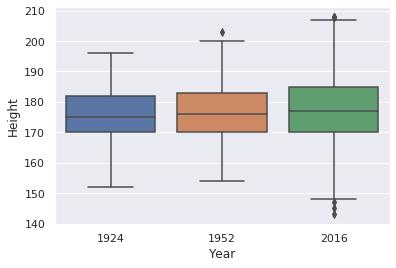In :
# Perform three two-sample t-tests
t_result_1924v2016 = stats.ttest_ind(athletes[athletes.Year == 1924].Height,
athletes[athletes.Year == 2016].Height)

t_result_1952v2016 = stats.ttest_ind(athletes[athletes.Year == 1952].Height,
athletes[athletes.Year == 2016].Height)

t_result_1924v1952 = stats.ttest_ind(athletes[athletes.Year == 1924].Height,
athletes[athletes.Year == 1952].Height)

# Create an array of p-value results
pvals_array = [t_result_1924v2016,t_result_1952v2016,t_result_1924v1952]
print('P-values:')
print(pd.DataFrame(pvals_array))

# Perform Bonferroni correction
print('\nCorrected P-values:')

P-values:
0
0  0.021908
1  0.022429
2  0.472911

Corrected P-values:
0
0  0.065725
1  0.067287
2  1.000000


## Šídák correction¶

We're looking at how the Height of Olympic athletes from the athletes dataset has changed over time. In this exercise, we're considering three events, the 100 meter, the High Jump, and the Marathon. We will be examining the correlation between Height and Year separately for each Event. As we did before, we will need to correct for multiple hypothesis tests, but, since these tests are independent, we can use the less-strict Šídák correction.

In :
fig, ax = plt.subplots(3,1, sharex=True, sharey=True, figsize=(8,6))

ax.scatter(x=athletes[athletes['Event'].isin(["Athletics Men's 100 metres",
"Athletics Women's 100 metres"])].Year,
y=athletes[athletes['Event'].isin(["Athletics Men's 100 metres",
"Athletics Women's 100 metres"])].Height,
color='red', s=10, alpha=0.5)

ax.scatter(x=athletes[athletes['Event'].isin(["Athletics Men's High Jump",
"Athletics Women's High Jump"])].Year,
y=athletes[athletes['Event'].isin(["Athletics Men's High Jump",
"Athletics Women's High Jump"])].Height,
color='lime', s=10, alpha=0.5)

ax.scatter(x=athletes[athletes['Event'].isin(["Athletics Men's Marathon",
"Athletics Women's Marathon"])].Year,
y=athletes[athletes['Event'].isin(["Athletics Men's Marathon",
"Athletics Women's Marathon"])].Height,
color='blue', s=10, alpha=0.5)
ax.set_xlabel('Date')
ax.set_title('100 Meter')
ax.set_title('High Jump')
ax.set_title('Marathon')

fig.text(0.001, 0.5, 'Height (meters)', va='center', rotation='vertical')
plt.tight_layout()
plt.show()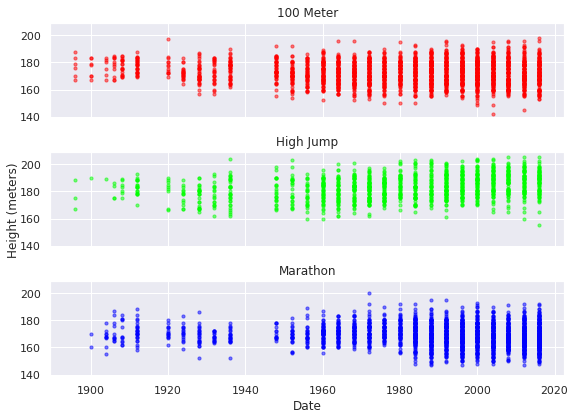In :
# Perform Pearson correlations
pearson100 = stats.pearsonr(athletes[athletes['Event'].isin(["Athletics Men's 100 metres",
"Athletics Women's 100 metres"])].Height,
athletes[athletes['Event'].isin(["Athletics Men's 100 metres",
"Athletics Women's 100 metres"])].Year)

pearsonHigh = stats.pearsonr(athletes[athletes['Event'].isin(["Athletics Men's High Jump",
"Athletics Women's High Jump"])].Height,
athletes[athletes['Event'].isin(["Athletics Men's High Jump",
"Athletics Women's High Jump"])].Year)

pearsonMara = stats.pearsonr(athletes[athletes['Event'].isin(["Athletics Men's Marathon",
"Athletics Women's Marathon"])].Height,
athletes[athletes['Event'].isin(["Athletics Men's Marathon",
"Athletics Women's Marathon"])].Year)

# Create array of p-values
pvals_array = [pearson100,pearsonHigh,pearsonMara]
print('P-values:')
print(pd.DataFrame(pvals_array))

# Perform Šídák correction
print('\nCorrected P-values:')

P-values:
0
0  1.170733e-03
1  4.934739e-23
2  3.336327e-08

Corrected P-values:
0
0  3.508088e-03
1  0.000000e+00
2  1.000898e-07


# Sample size and power¶

The power of a binary hypothesis test is the probability that the test rejects the null hypothesis (H0) when a specific alternative hypothesis (H1) is true. The statistical power ranges from 0 to 1, and as statistical power increases, the probability of making a type II error (failing to find difference that does exist) decreases. For a type II error probability of β, the corresponding statistical power is 1 − β. For example, if experiment 1 has a statistical power of 0.7, and experiment 2 has a statistical power of 0.95, then there is a stronger probability that experiment 1 had a type II error than experiment 2, and experiment 2 is more reliable than experiment 1 due to the reduction in probability of a type II error. It can be equivalently thought of as the probability of accepting the alternative hypothesis (H1) when it is true—that is, the ability of a test to detect a specific effect, if that specific effect actually exists. That is,

$$power = Pr \: ( reject \: H_o \: | \: H_1 \: is \: true)$$
• Low Statistical Power: Large risk of committing Type II errors, e.g. a false negative.
• High Statistical Power: Less risk of committing Type II errors.
• A power analysis can be used to estimate the minimum sample size required for an experiment, given a desired significance level, effect size, and statistical power.

Power Analysis:

• Effect Size: The quantified magnitude of a result present in the population. Effect size is calculated using a specific statistical measure, such as Pearson’s correlation coefficient for the relationship between variables or Cohen’s d for the difference between groups.
• Sample Size: The number of observations in the sample. Larger sample size = more sensitive methods.
• Significance: The significance level used in the statistical test, e.g. alpha. Often set to 5% or0.05.
• Statistical Power: The probability of accepting the alternative hypothesis if it is true.

Measures of effect size:

• Cohen's d:
• Continuous variables in relation to discrete variables.
• Normalized differences between the means of two samples.
• Odds ratio:
• For discrete variables.
• How much one event is associated with another.
• Correlation coefficients:
• For continuous variables.
• Measures correlation.

For a given experiment with Significance level (alpha): 5% or 0.05, Effect Size: of at least 0.80, Statistical Power: 80% or 0.80. We may be interested in estimating a suitable sample size. That is, how many observations are required from each sample in order to at least detect an effect of 0.80 with an 80% chance of detecting the effect if it is true (20% of a Type II error) and a 5% chance of detecting an effect if there is no such effect (Type I error).

The function solve_power() can be used to calculate one of the four parameters in a power analysis. In our case, we are interested in calculating the sample size. We can use the function by providing the three pieces of information we know (alpha, effect, and power) and setting the size of argument we wish to calculate the answer of (nobs1) to None. This tells the function what to calculate.

The function has an argument called ratio that is the ratio of the number of samples in one sample to the other. If both samples are expected to have the same number of observations, then the ratio is 1.0. If, for example, the second sample is expected to have half as many observations, then the ratio would be 0.5.

## Exploring sample size¶

Now we'll explore the effect of sample size on the results of statistical tests. Here, we'll be comparing the Weight of American Athletics and Swimming competitors in the athletes dataset. The boxplot show the difference between these two groups.

In :
sns.boxplot(x="Sport", y="Weight", data=athletes);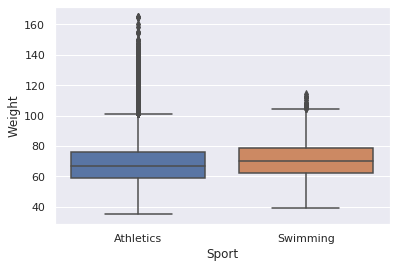Using a defined seed and varying sample sizes, we will perform t-tests comparing the Weight of samples from both Sports.

In :
# Create a random subset of 1000 samples, then use this to do a t-test to compare Weight between Sports.
subset = athletes.sample(n=1000, random_state= 1007)
ttestind = stats.ttest_ind(subset[subset.Sport == "Athletics"].Weight, subset[subset.Sport == "Swimming"].Weight)
print(f'Test Statistic = {round(ttestind,2)}, P-value = {round(ttestind,2)}')

Test Statistic = -1.84, P-value = 0.07

In :
# Change the sample size to 200, repeat the t-test to compare Weight between Sports.
subset = athletes.sample(n=200, random_state= 1007)
ttestind = stats.ttest_ind(subset[subset.Sport == "Athletics"].Weight, subset[subset.Sport == "Swimming"].Weight)
print(f'Test Statistic = {round(ttestind,2)}, P-value = {round(ttestind,2)}')

Test Statistic = -2.89, P-value = 0.0

In :
# Change the sample size to 50, repeat the t-test to compare Weight between Sports.
subset = athletes.sample(n=50, random_state= 1007)
ttestind = stats.ttest_ind(subset[subset.Sport == "Athletics"].Weight, subset[subset.Sport == "Swimming"].Weight)
print(f'Test Statistic = {round(ttestind,2)}, P-value = {round(ttestind,2)}')

Test Statistic = -0.57, P-value = 0.57


Look how smaller samples affected your p-value. With sample sizes of 1000, 200 and 50, we got p-values of 0.07, 0.0 and 0.57, respectively. The smallest sample size didn't give a significant result. Now you see how much difference sample size can make.

## Sample size for a t-test¶

Now that we've seen the importance of sample size, let's have another look at the same athletes dataset and see if we can determine the sample size we would need to get a significant result. We will determine the sample size we would need to have an 80% chance of detecting a small (0.4) difference between these two samples.

In :
from statsmodels.stats import power as pwr

# Set parameters
effect = 0.4
power = 0.8
alpha = 0.05

# Calculate ratio
swimmercount = float(len(athletes[athletes.Sport == "Swimming"].index))
athletecount = float(len(athletes[athletes.Sport == "Athletics"].index))
ratio = swimmercount/athletecount

# Initialize analysis and calculate sample size
analysis = pwr.TTestIndPower()
ssresult = analysis.solve_power(effect_size=effect, power=power, alpha=alpha, nobs1=None, ratio=ratio)

print(f'Sample Size: {round(ssresult,3)}')

Sample Size: 134.864


## Effect size for a t-test¶

Now, we're going to have a look at effect sizes. We are examining the same slice from the atheletes dataset, comparing the body weights of competitors from swimming and athletics events. We are going to calculate the effect sizes we are able to detect for a couple of different sampling strategies. First, we will determine the smallest effect size detectable using the complete dataset. Then, in Step 2, we will determine the size effect we could detect using 300 samples from each Sport.

In :
# Set parameters for entire dataset
alpha = 0.05
power = 0.8
ratio = float(len(athletes[athletes.Sport == "Swimming"])) / len(athletes[athletes.Sport == "Athletics"])
samp_size = len(athletes[athletes.Sport == "Athletics"])

# Initialize analysis & calculate effect size
analysis = pwr.TTestIndPower()
esresult = analysis.solve_power(effect_size = None,
power = power,
nobs1 = samp_size,
ratio = ratio,
alpha = alpha)

print(f'Minimum detectable effect size: {round(esresult,2)}')

Minimum detectable effect size: 0.03

In :
# Set parameters for the sample size found from the power calculation
alpha = 0.05
power = 0.8
ratio = float(len(athletes[athletes.Sport == "Swimming"])) / len(athletes[athletes.Sport == "Athletics"])
samp_size = round(ssresult,0)

# Initialize analysis & calculate sample size
analysis = pwr.TTestIndPower()
esresult = analysis.solve_power(effect_size=None, power=power, nobs1=samp_size, ratio=ratio, alpha=alpha)

print(f'Minimum detectable effect size: {round(esresult,2)}')

Minimum detectable effect size: 0.4


## Cohen's d¶

Cohen's d is an effect size used to indicate the standardised difference between two means. It can be used, for example, to accompany reporting of t-test and ANOVA results.For the independent samples T-test, Cohen's d is determined by calculating the mean difference between your two groups, and then dividing the result by the pooled standard deviation.

### Computing Cohen's d¶

Now, using the same comparison of Weight difference between Sports, let's calculate the actual effect size for this comparison. We will calculate Cohen's d for the difference in Weight between each Sport.

In :
# sebset DataFrame with sample size found from the power calculation
subset = athletes.sample(n=int(round(ssresult,0)), random_state=2020)

# Create series
athl = athletes[athletes.Sport == "Athletics"].Weight
swim = athletes[athletes.Sport == "Swimming"].Weight

# Calculate difference between means and pooled standard deviation
diff = swim.mean() - athl.mean()
pooledstdev = np.sqrt((athl.std()**2 + swim.std()**2)/2)

# Calculate Cohen's d
cohend = diff / pooledstdev

print(f"Cohen's d: {round(cohend,3)}")

Cohen's d: 0.098


This is a moderate sized effect size. As the effect size is bigger than the minimum effect size calculated in the last exercise, you would expect that the parameters from the last exercise would allow you to detect a difference similar to this output.

## Effect size for a Fisher exact test¶

In this exercise, we will use the athletes dataset to examine whether American athletes are more successful in athletics or in swimming events. We will make use of the MedalTF column, which gives a True or False value for whether each athlete won any type of medal. First, we will cross-tabulate MedalTF with Sport. Then perform a Fisher exact test. Finally, we will examine the significance and effect size of this result.

In :
# Create a table of cross-tabulations
table = pd.crosstab(athletes.MedalTF,athletes.Sport)
print(table)

# Perform the Fisher exact test
chi = stats.fisher_exact(table, alternative='two-sided')

# Print p-value
print(f"\np-value: {round(chi, 5)}")

# Print odds ratio
print(f"\nOdds ratio between groups: {round(chi,1)}")

Sport    Athletics  Swimming
MedalTF
False        28726     16290
True          3648      2486

p-value: 0.0

Odds ratio between groups: 1.2


## Effect sizes for Pearson correlation¶

Now we're going to look at effect sizes for Pearson correlations. In a previous exercise, we used Pearson correlation to examine the link between Weight and Height for athletes from our Olympic dataset. Now, we're going to focus a single event, the 10,000 meter run, and compare the results we obtain for Pearson tests of correlation for competitors from two Teams, Kenya (ken DataFrame) and Ethiopia (eth DataFrame). Which has a higher effect strength?

In :
ken = athletes[athletes['Team'] == "Kenya"]
eth = athletes[athletes['Team'] == "Ethiopia"]

fig, ax = plt.subplots(2,1, sharex=True, sharey=True, figsize=(8,6))

ax.scatter(x=ken[ken['Event'].isin(["Athletics Men's 10,000 metres",
"Athletics Women's 10,000 metres"])].Height,
y=ken[ken['Event'].isin(["Athletics Men's 10,000 metres",
"Athletics Women's 10,000 metres"])].Weight,
color='red', s=25)

ax.scatter(x=eth[eth['Event'].isin(["Athletics Men's 10,000 metres",
"Athletics Women's 10,000 metres"])].Height,
y=eth[eth['Event'].isin(["Athletics Men's 10,000 metres",
"Athletics Women's 10,000 metres"])].Weight,
color='blue', s=25)

ax.set_xlabel('Height')
ax.set_title('Kenya 10,000 metres')
ax.set_title('Ethiopia 10,000 metres')

fig.text(0.001, 0.5, 'Weight', va='center', rotation='vertical')
plt.tight_layout()
plt.show()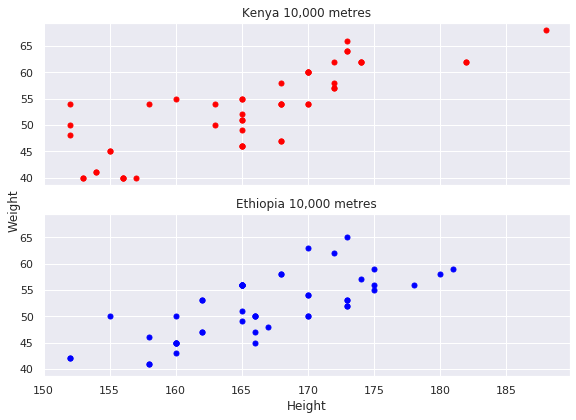In :
# Perform Pearson correlation Kenya
pearsonken = stats.pearsonr(ken[ken['Event'].isin(["Athletics Men's 10,000 metres",
"Athletics Women's 10,000 metres"])].Weight,
ken[ken['Event'].isin(["Athletics Men's 10,000 metres",
"Athletics Women's 10,000 metres"])].Height)

print(f'Kenya Correlation: {round(pearsonken,3)}, P-value: {round(pearsonken,3)}')

Kenya Correlation: 0.821, P-value: 0.0

In :
# Perform Pearson correlation Ethiopia
pearsoneth = stats.pearsonr(eth[eth['Event'].isin(["Athletics Men's 10,000 metres",
"Athletics Women's 10,000 metres"])].Weight,
eth[eth['Event'].isin(["Athletics Men's 10,000 metres",
"Athletics Women's 10,000 metres"])].Height)

print(f'Ethiopia Correlation: {round(pearsoneth,3)}, P-value: {round(pearsoneth,3)}')

Ethiopia Correlation: 0.718, P-value: 0.0


The Kenyan dataset gives a higher value than that obtained for the Ethiopian samples. This makes sense, as the scatterplot for the former looks much less noisy.

## Power analysis for a t-test¶

Now we're going to have another look at the same example, drawn from our Olympic dataset (Weight difference between Sports). Knowing the effect size of the difference between these two groups, we will work out what the odds are that our t-test will pick up this difference.

In :
# Set parameters
effect_size = esresult
alpha = 0.05
samp_size = round(ssresult,0)
ratio = 1

# Initialize analysis & calculate power
analysis = pwr.TTestIndPower()
pwresult = analysis.solve_power(effect_size=effect_size, power=None, alpha=alpha, nobs1=samp_size, ratio=ratio)

print(f'Power: {round(pwresult,3)}')

Power: 0.905


### Power curves¶

Power curves are line plots that show how the change in variables, such as effect size and sample size, impact the power of the statistical test.

The plot_power() function can be used to create power curves. The dependent variable (x-axis) must be specified by name in the ‘dep_var‘ argument. Arrays of values can then be specified for the sample size (nobs), effect size (effect_size), and significance (alpha) parameters. One or multiple curves will then be plotted showing the impact on statistical power.

In :
# parameters for power analysis
effect_sizes = np.array([0.1, 0.3, 0.4, 0.5, 0.6, 0.7, 0.8, 0.95])
sample_sizes = np.array(range(2, 151))
sample_sizes0 = np.array([10, 20, 30, 40, 50, 60, 70, 100])

# calculate power curves from multiple power analyses
analysis = pwr.TTestIndPower()

fig, ax = plt.subplots(2,1, figsize=(10,7))
analysis.plot_power(dep_var='nobs', nobs=sample_sizes, effect_size=effect_sizes, alpha=0.05, ax=ax)
analysis.plot_power(dep_var='es', nobs=sample_sizes0, effect_size=effect_sizes, alpha=0.05, ax=ax)
ax.set_title('')
ax.legend(bbox_to_anchor=(1, 1.05))
ax.legend(bbox_to_anchor=(1, 1.05))
ax.margins(0.015)
ax.margins(0.015)
fig.text(0.001, 0.5, 'Power', va='center', rotation='vertical')
plt.tight_layout()
plt.show()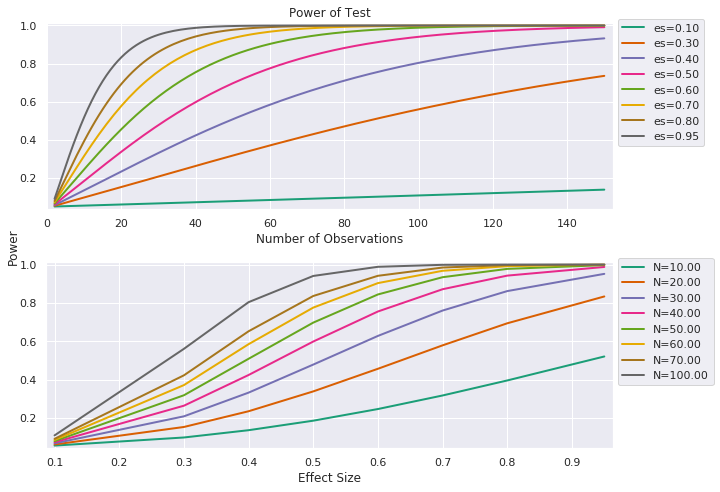# Testing for normality¶

Shapiro-Wilk test:

The Shapiro-Wilk test is a way to tell if a set of observations comes from a normal distribution. The test gives you a $W$ value; small values indicate your sample is not normally distributed (you can reject the null hypothesis that your population is normally distributed if your values are under a certain threshold).

• If the p value is less than the chosen alpha level, then the null hypothesis is rejected and there is evidence that the data tested are not normally distributed.
• If the p value is greater than the chosen alpha level, then the null hypothesis that the data came from a normally distributed population cannot be rejected .
In :
pd.set_option('display.max_columns', None)
countrydata.info()

<class 'pandas.core.frame.DataFrame'>
RangeIndex: 229 entries, 0 to 228
Data columns (total 50 columns):
country                                                       229 non-null object
Region                                                        229 non-null object
Surface area (km2)                                            226 non-null object
Population in thousands (2017)                                229 non-null int64
Population density (per km2, 2017)                            229 non-null float64
Sex ratio (m per 100 f, 2017)                                 227 non-null float64
GDP: Gross domestic product (million current US$) 208 non-null float64 GDP growth rate (annual %, const. 2005 prices) 208 non-null object GDP per capita (current US$)                                  208 non-null float64
Economy: Agriculture (% of GVA)                               206 non-null object
Economy: Industry (% of GVA)                                  208 non-null float64
Economy: Services and other activity (% of GVA)               208 non-null float64
Employment: Agriculture (% of employed)                       210 non-null object
Employment: Industry (% of employed)                          211 non-null object
Employment: Services (% of employed)                          211 non-null object
Unemployment (% of labour force)                              218 non-null object
Labour force participation (female/male pop. %)               215 non-null object
Agricultural production index (2004-2006=100)                 211 non-null float64
Food production index (2004-2006=100)                         211 non-null float64
International trade: Exports (million US$) 211 non-null object International trade: Imports (million US$)                    211 non-null object
International trade: Balance (million US$) 211 non-null object Balance of payments, current account (million US$)            187 non-null object
Population growth rate (average annual %)                     229 non-null object
Urban population (% of total population)                      229 non-null float64
Urban population growth rate (average annual %)               229 non-null object
Fertility rate, total (live births per woman)                 218 non-null object
Life expectancy at birth (females/males, years)               217 non-null object
Population age distribution (0-14 / 60+ years, %)             226 non-null object
International migrant stock (000/% of total pop.)             229 non-null object
Refugees and others of concern to UNHCR (in thousands)        188 non-null object
Infant mortality rate (per 1000 live births                   206 non-null object
Health: Total expenditure (% of GDP)                          190 non-null float64
Health: Physicians (per 1000 pop.)                            190 non-null object
Education: Government expenditure (% of GDP)                  184 non-null object
Education: Primary gross enrol. ratio (f/m per 100 pop.)      196 non-null object
Education: Secondary gross enrol. ratio (f/m per 100 pop.)    192 non-null object
Education: Tertiary gross enrol. ratio (f/m per 100 pop.)     180 non-null object
Seats held by women in national parliaments %                 192 non-null float64
Mobile-cellular subscriptions (per 100 inhabitants)           216 non-null object
Mobile-cellular subscriptions (per 100 inhabitants).1         213 non-null object
Individuals using the Internet (per 100 inhabitants)          228 non-null float64
Threatened species (number)                                   221 non-null object
Forested area (% of land area)                                216 non-null object
CO2 emission estimates (million tons/tons per capita)         209 non-null float64
Energy production, primary (Petajoules)                       219 non-null float64
Energy supply per capita (Gigajoules)                         209 non-null object
Pop. using improved drinking water (urban/rural, %)           208 non-null object
Pop. using improved sanitation facilities (urban/rural, %)    142 non-null object
Net Official Development Assist. received (% of GNI)          0 non-null float64
dtypes: float64(15), int64(1), object(34)
memory usage: 89.6+ KB

In :
countrydata['Unemployment (% of labour force)'].replace('...', np.nan, inplace=True)
countrydata['Unemployment (% of labour force)'] = countrydata['Unemployment (% of labour force)'].astype(float)


## Shapiro-Wilk test¶

Now, we will look at at country-level Unemployment and GDP per capita (GDP_per_cap) data. We will use a Shapiro-Wilk test to examine whether the distribution of values seen in these samples, as seen in the Q-Q plots below, departs significantly from the normal distribution. This test tells us how closely a given sample fits the patterns expected from a normal distribution.

In :
from scipy.stats import probplot
import warnings
warnings.filterwarnings('ignore')

fig, ax = plt.subplots(2,2, figsize=(10,7))

sns.kdeplot(countrydata['Unemployment (% of labour force)'].dropna(), ax=ax, color='red', shade=True, legend=False)
sns.kdeplot(countrydata['GDP per capita (current US$)'].dropna(), ax=ax, color='blue', shade=True, legend=False) probplot(countrydata['Unemployment (% of labour force)'].dropna(), plot=ax) probplot(countrydata['GDP per capita (current US$)'].dropna(), plot=ax)

ax.set_title('Unemployment (% of labour force)')
ax.set_title('GDP per capita (current US$)') ax.set_title('') ax.set_title('') plt.tight_layout() plt.show()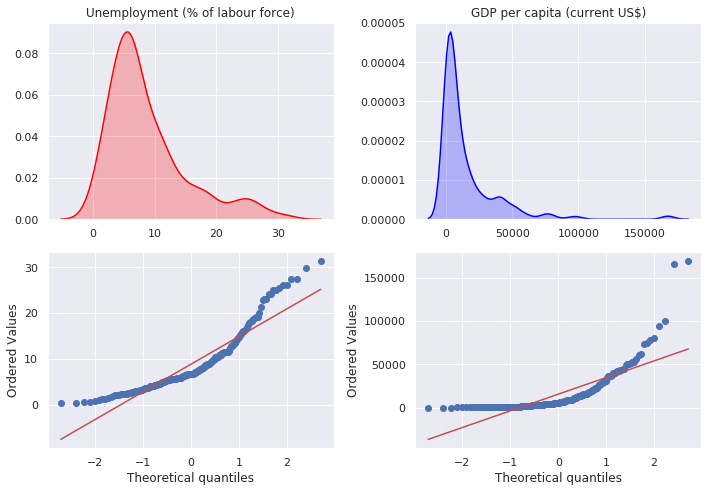In : # Perform Shapiro-Wilk test on Unemployment and print result shapiroUnem = stats.shapiro(countrydata['Unemployment (% of labour force)'].dropna()) print(f'W: {round(shapiroUnem,3)}, P-value: {round(shapiroUnem,3)}')  W: 0.868, P-value: 0.0  In : # Perform Shapiro-Wilk test on GDP_per_cap and print result shapiroGDP = stats.shapiro(countrydata['GDP per capita (current US$)'].dropna())

print(f'W: {round(shapiroGDP,3)}, P-value: {round(shapiroGDP,3)}')

W: 0.63, P-value: 0.0


Both distributions are non-normal.

## D’Agostino’s K^2 Test¶

Tests whether a data sample has a Gaussian distribution. The test is based on transformations of the sample kurtosis and skewness, and has power only against the alternatives that the distribution is skewed and/or kurtic.

Interpretation:

• H0: the sample has a Gaussian distribution.
• H1: the sample does not have a Gaussian distribution.
In :
# Perform D'Agostino's K^2 test on Unemployment and print result
k2_Unem, k2_Unem_p = stats.normaltest(countrydata['Unemployment (% of labour force)'].dropna())

print('stat = %.3f, p_val = %.3f' % (k2_Unem, k2_Unem_p))

if k2_Unem_p >= 0.05:
print('\nProbably Gaussian')
else:
print('\nProbably not Gaussian')

stat = 50.735, p_val = 0.000

Probably not Gaussian

In :
# Perform D'Agostino's K^2 test on GDP_per_cap and print result
k2_GDP, k2_GDP_p = stats.normaltest(countrydata['GDP per capita (current US$)'].dropna()) print('stat = %.3f, p_val = %.3f' % (k2_GDP, k2_GDP_p)) if k2_GDP_p >= 0.05: print('\nProbably Gaussian') else: print('\nProbably not Gaussian')  stat = 176.387, p_val = 0.000 Probably not Gaussian  ## Anderson-Darling Test¶ The Anderson-Darling Goodness of Fit Test (AD-Test) is a measure of how well your data fits a specified distribution. It’s commonly used as a test for normality. Assumptions: • H0: the sample has a Gaussian distribution. • H1: the sample does not have a Gaussian distribution. In : # Example of the Anderson-Darling Normality Test data = countrydata['GDP per capita (current US$)'].dropna()
result = stats.anderson(data)

print('stat=%.3f' % (result.statistic))

for i in range(len(result.critical_values)):
sl, cv = result.significance_level[i], result.critical_values[i]
if result.statistic < cv:
print('\nProbably Gaussian at the %.1f%% level' % (sl))
else:
print('\nProbably not Gaussian at the %.1f%% level' % (sl))

stat=22.048

Probably not Gaussian at the 15.0% level

Probably not Gaussian at the 10.0% level

Probably not Gaussian at the 5.0% level

Probably not Gaussian at the 2.5% level

Probably not Gaussian at the 1.0% level

In :
# Example of the Anderson-Darling Normality Test
data = countrydata['Unemployment (% of labour force)'].dropna()
result = stats.anderson(data)

print('stat=%.3f' % (result.statistic))

for i in range(len(result.critical_values)):
sl, cv = result.significance_level[i], result.critical_values[i]
if result.statistic < cv:
print('\nProbably Gaussian at the %.1f%% level' % (sl))
else:
print('\nProbably not Gaussian at the %.1f%% level' % (sl))

stat=8.507

Probably not Gaussian at the 15.0% level

Probably not Gaussian at the 10.0% level

Probably not Gaussian at the 5.0% level

Probably not Gaussian at the 2.5% level

Probably not Gaussian at the 1.0% level


# Parametric vs. Non-parametric tests¶

A parametric statistical test makes an assumption about the population parameters and the distributions that the data came from. These types of test includes Student’s T tests and ANOVA tests, which assume data is from a normal distribution.

The opposite is a nonparametric test, which doesn’t assume anything about the population parameters. Nonparametric tests include chi-square, Fisher’s exact test and the Mann-Whitney test.

Every parametric test has a nonparametric equivalent. For example, if you have parametric data from two independent groups, you can run a 2 sample t test to compare means. If you have nonparametric data, you can run a Wilcoxon rank-sum test to compare means.

Wilcoxon rank-sum (Mann–Whitney U test):

• Non-parametric
• Not sensitive to distribution shape.

The Wilcoxon rank-sum test is a nonparametric test of the null hypothesis that it is equally likely that a randomly selected value from one population will be less than or greater than a randomly selected value from a second population. This test can be used to investigate whether two independent samples were selected from populations having the same distribution.

Wilcoxon signed-rank:

• Non-parametric equivalent to paired t-test
• Tests if ranks differ across pairs

The Wilcoxon signed-rank test is a non-parametric statistical hypothesis test used to compare two related samples, matched samples, or repeated measurements on a single sample to assess whether their population mean ranks differ (i.e. it is a paired difference test). It can be used as an alternative to the paired Student's t-test when the distribution of the differences between the two samples cannot be assumed to be normally distributed. A Wilcoxon signed-rank test is a nonparametric test that can be used to determine whether two dependent samples were selected from populations having the same distribution.

## Wilcoxon rank-sum test (Mann–Whitney U test)¶

Here we have the sex ratio of European Union and the United States (US_Sex_ratio) with the sex ratio of Asian countries (EU_Sex_ratio).

In :
gender = pd.read_csv('sex-ratio-at-birth.csv')
gender.columns = ['Country', 'Code', 'Year', 'Sex_ratio']

Out:
Country Code Year Sex_ratio
0 Afghanistan AFG 1962 106.0
1 Afghanistan AFG 1967 106.0
2 Afghanistan AFG 1972 106.0
3 Afghanistan AFG 1977 106.0
4 Afghanistan AFG 1982 106.0
In :
US_Sex_ratio = gender[gender.Country == "United States"].Sex_ratio
EU_Sex_ratio = gender[gender.Country == "European Union"].Sex_ratio

plt.legend(('United States','European Union'))
plt.show()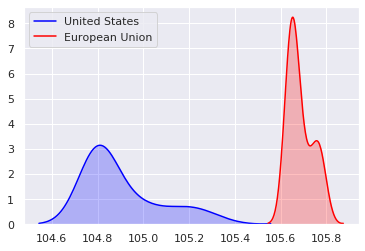Given that these samples are not normally distributed, a Wilcoxon rank-sum test would be appropriate. Does the sex ratio differ between countries?

In :
# Perform the two-sample t-test
t_result = stats.ttest_ind(US_Sex_ratio, EU_Sex_ratio)

# Print t-test result
print(f'Test Statistic = {t_result}, P-value = {t_result}')

# Perform Wilcoxon rank-sum test
wilc = stats.ranksums(US_Sex_ratio, EU_Sex_ratio)

# Print Wilcoxon rank-sum testresult
print(f'\nTest Statistic = {wilc}, P-value = {wilc}')

Test Statistic = -20.468097438530254, P-value = 1.8277144237715248e-22

Test Statistic = -5.477225575051661, P-value = 4.320463057827488e-08


## Wilcoxon signed-rank test¶

We are interested in potato yield in tons/hectare, as seen in previous exercises. For each Field, we have a value for Yield2018, before the application of a new fertilizer, and Yield2019, after the application of the new fertilizer. Does the yield differ significantly between years?

In :
yields2018 = [60.2, 12.0, 13.8, 91.8, 50.0, 45.0, 32.0, 87.5, 60.1, 88.0, 11.3, 10.7, 12.7, 15.9, 16.0]
yields2019 = [63.2, 15.6, 14.8, 96.7, 53.0, 47.0, 31.3, 89.8, 67.8, 90.0, 14.2, 12.0, 13.9, 17.8, 20.0]

podataframe = pd.DataFrame({'Yield2018': yields2018,
'Yield2019' : yields2019})
print(podataframe)

    Yield2018  Yield2019
0        60.2       63.2
1        12.0       15.6
2        13.8       14.8
3        91.8       96.7
4        50.0       53.0
5        45.0       47.0
6        32.0       31.3
7        87.5       89.8
8        60.1       67.8
9        88.0       90.0
10       11.3       14.2
11       10.7       12.0
12       12.7       13.9
13       15.9       17.8
14       16.0       20.0

In :
fig, ax = plt.subplots(nrows=1, ncols=2, figsize=(12,5))
sns.boxplot(data=podataframe, ax=ax)
ax.legend(('Yield2018', 'Yield2019'))
ax.set_xlabel('Yeild')
plt.tight_layout()
plt.show()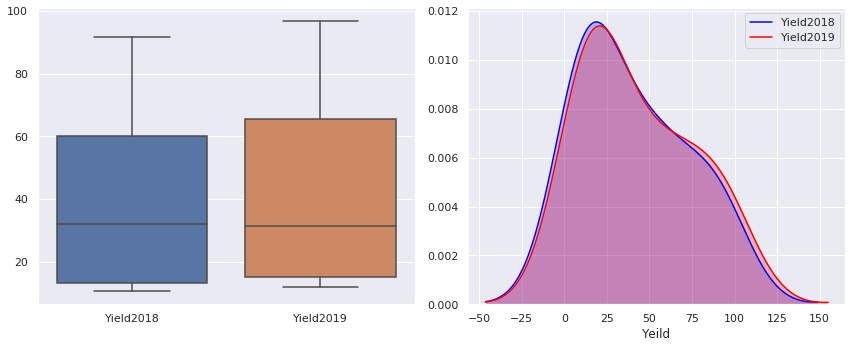In :
# Perform the two-sample t-test
t_result = stats.ttest_ind(podataframe.Yield2018, podataframe.Yield2019)
print(f'T-Test Test Statistic = {t_result}, P-value = {t_result}')

# Perform Shapiro-Wilk test on Yield2018 and print result
shapiroYield2018 = stats.shapiro(podataframe.Yield2018)
print(f'\nYield2018 W: {round(shapiroYield2018,3)}, P-value: {round(shapiroYield2018,3)}')

# Perform Shapiro-Wilk test on Yield2019 and print result
shapiroYield2019 = stats.shapiro(podataframe.Yield2019)
print(f'\nYield2019 W: {round(shapiroYield2019,3)}, P-value: {round(shapiroYield2019,3)}')

# Perform Wilcoxon Signed-Rank test
wilcsr = stats.wilcoxon(podataframe.Yield2018, podataframe.Yield2019)
print(f'\nWilcoxon Signed-Rank Test Statistic = {wilcsr}, P-value = {wilcsr}')

T-Test Test Statistic = -0.23488929598202465, P-value = 0.816003972907755

Yield2018 W: 0.836, P-value: 0.011

Yield2019 W: 0.844, P-value: 0.014

Wilcoxon Signed-Rank Test Statistic = 1.0, P-value = 0.0001220703125


Note that both of your Shapiro-Wilks tests gave significant results, indicating non-normal distributions. The Wilcoxon test gives a higher p-value for the same data, due to its lower sensitivity. However, due to the low sample sizes and non-normal distributions, the Wilcoxon test is a better choice of test for this dataset.

## Parametric vs non-parametric tests¶

Once again we'll be using the Olympic dataset. Here we're going to compare the Height of athletes from both the Norwegian and Chinese Teams. Do they differ?

In :
# Separate the heights by country
NorwayHeights = athletes[athletes['Team'] == "Norway"].Height
ChinaHeights = athletes[athletes['Team'] == "China"].Height